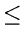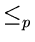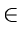Next:10.5.1 NP-Hardness and NP-CompletenessUp:10. Introduction to NP-CompletenessPrevious:10.4 The Classes P and NP

# 10.5 NP-Complete Problems

The definition of NP-completeness is based on reducibility of problems. Suppose we wish to solve a problem X and we already have an algorithm for solving another problem Y. Suppose we have a function T that takes an input x for X and produces T(x), an input for Y such that the correct answer for X on x is yes if and only if the correct answer for Y on T(x) is yes. Then by composing T and the algorithm for y, we have an algorithm for X.
• If the function T itself can be computed in polynomially bounded time, we say X is polynomially reducible to Y and we write Xp Y.
• If X is polynomially reducible to Y, then the implication is that Y is at least as hard to solve as X. i.e. X is no harder to solve than Y.
• It is easy to see that

•Y and YP implies XP.

eEL,CSA_Dept,IISc,Bangalore